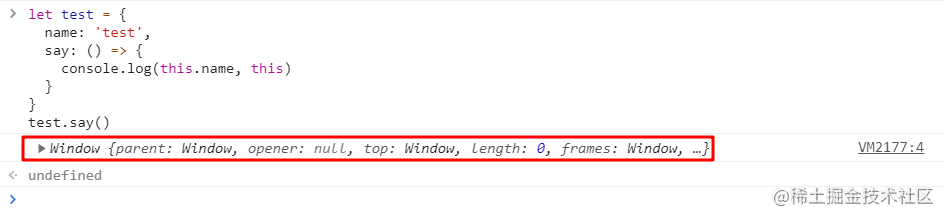# ES6 Function 之箭头函数

## 0. 前言

ES6 中的箭头函数长这样：`()=>{}`

## 1. `ES6` 之前声明函数的做法

``````// 方式 1：函数声明
function hello1 () {}
// 方式 2：函数表达式，又叫函数字面量
let hello2 = function () {}
// 方式 3：函数构造法，参数必须加引号
let hello3 = new Function()

## 2. `ES6` 中使用箭头函数声明函数

### 2.1 箭头函数的声明

``````// 声明一个函数，函数名为 hello
let hello = () => { // 注意：小括号不能省略哦
console.log('hello world')
}
// 调用这个函数
hello() // hello world

``````// 声明一个函数，函数名为 hello，可以接收一个 name 参数
let hello = (name) => {
console.log('hello world', name)
}
hello('jjLin') // hello world jjLin

``````let hello = (name, city) => {
console.log('hello world', name, city)
}
hello('jjLin', 'shanghai') // hello world jjLin shanghai

### 2.2 箭头函数声明时的省略与简写

``````let hello = name => {
console.log('hello world', name)
}
hello('jjLin') // hello world jjLin

``````let sum = (x, y, z) => x + y + z // x + y + z 是一个表达式，这个表达式的值就是函数的返回值
console.log(sum(1, 2, 3)) // 6

``````var func = () => { foo: 1 };
// Calling func() returns undefined!

var func = () => { foo: function() {} };
// SyntaxError: function statement requires a name

let sum = (x, y, z) => {
x: x,
y: y,
z: z
} // Uncaught SyntaxError: Unexpected token ':'

``````var func = () => ({foo: 1});

let sum = (x, y, z) => ({
x: x,
y: y,
z: z
})
console.log(sum(1, 2, 3)) // {x: 1, y: 2, z: 3}

``````let sum = (x, y, z) => {
return {
x: x,
y: y,
z: z
}
}
console.log(sum(1, 2, 3)) // {x: 1, y: 2, z: 3}

## 3. 箭头函数没有单独的 `this`

• 如果是该函数是一个构造函数，`this` 指针指向一个新的对象
• 在严格模式下的函数调用下，`this` 指向 `undefined`
• 如果是该函数是一个对象的方法，则它的 `this` 指针指向这个对象
• 等等

`this` 被证明是令人厌烦的面向对象风格的编程。

``````function Person () {
// Person() 构造函数定义 `this` 作为它自己的实例.
this.age = 0

setInterval(function growUp () {
// 在非严格模式, growUp() 函数定义 `this` 作为全局对象,
// 与在 Person() 构造函数中定义的 `this` 并不相同.
this.age++
}, 1000)
}

var p = new Person()

`ESMAScript 3/5` 中，通过将 `this` 值分配给封闭的变量，可以解决 `this` 问题。

``````function Person () {
var that = this
that.age = 0

setInterval(function growUp () {
// 回调引用的是`that`变量, 其值是预期的对象.
that.age++
}, 1000)
}

``````function Person () {
this.age = 0

setInterval(() => {
this.age++ // this 正确地指向 p 实例
}, 1000)
}

var p = new Person()

``````let test = {
name: 'test',
say: function () {
console.log(this.name)
}
}
test.say() // test

`test.say()` 的结果是什么？

``````let test = {
name: 'test',
say: () => {
console.log(this.name, this)
}
}
test.say() // undefined {}